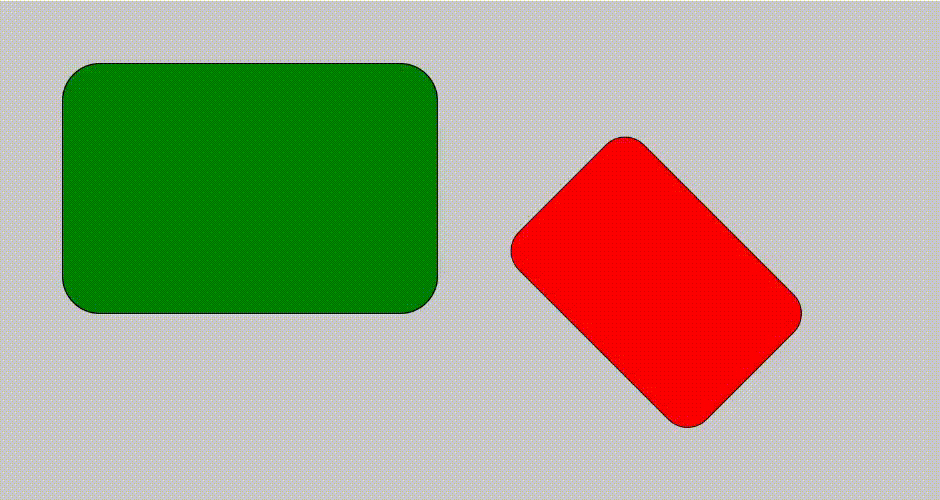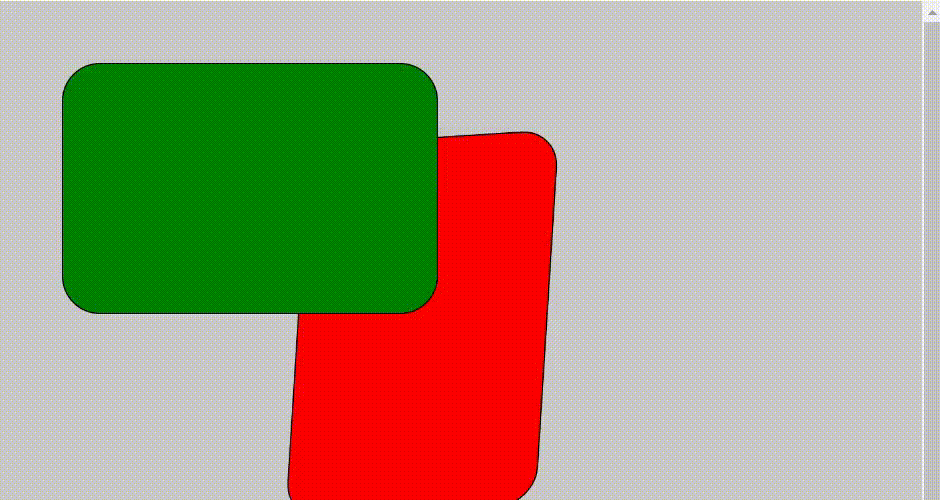# p5.js resetMatrix() Function

• Difficulty Level : Medium
• Last Updated : 01 Apr, 2021

The resetMatrix() function is used to replace the current matrix with the identity matrix (The square matrix whose all values are zero except diagonal which is 1). When we are rotating, translating, and scaling any graphics image using applyMatrix()  then by applying the function resetMatrix() we can change the graphics to its original form.

Syntax:

Hey geek! The constant emerging technologies in the world of web development always keeps the excitement for this subject through the roof. But before you tackle the big projects, we suggest you start by learning the basics. Kickstart your web development journey by learning JS concepts with our JavaScript Course. Now at it's lowest price ever!

`resetMatrix()`

Below are the examples to illustrate the resetMatrix() function.

Step 1: Open online web editor https://editor.p5js.org/.

Step 2: Write the following code and run to see the output.

Example 1:

## Javascript

 `// Set up the function``function` `setup() {`` ` `    ``// Create canvas``    ``createCanvas(800, 400);``}`` ` `function` `draw() {`` ` `    ``// Set the background colour``    ``background(200);`` ` `    ``// Set the translate function``    ``translate(500, 50);`` ` `    ``// Set the apply matrix function``    ``applyMatrix(0.5, 0.5, -0.5, 0.5, 0, 0);`` ` `    ``// Set the colour to fill the graphics``    ``fill(``'red'``);`` ` `    ``// Set the shape.``    ``rect(50, 50, 300, 200, 30);`` ` `    ``// Now call the reset function``    ``resetMatrix();`` ` `    ``// Set the colour to fill the graphics``    ``fill(``'green'``);`` ` `    ``// Set the shape``    ``rect(50, 50, 300, 200, 30);``}`

Output:Example 2:

## Javascript

 `// Set up the function``function` `setup() {`` ` `    ``// Create canvas``    ``createCanvas(800, 600);``}`` ` `function` `draw() {`` ` `    ``// Set the function to rotate``    ``let step = frameCount % 50;``    ``let angle = map(step, 10, 60, 0, PI);``    ``let cos_a = cos(angle);``    ``let sin_a = sin(angle);`` ` `    ``// Set the background color``    ``background(200);`` ` `    ``// Set the translate function``    ``translate(500, 50);`` ` `    ``// Set the apply matrix function``    ``applyMatrix(cos_a, sin_a, -sin_a, -cos_a, 0, 0);`` ` `    ``// Set the colour to fill the graphics``    ``fill(``'red'``);`` ` `    ``// Set the shape``    ``rect(50, 50, 300, 200, 30);`` ` `    ``// Now call the reset function``    ``resetMatrix();`` ` `    ``// Set the colour to fill the graphics``    ``fill(``'green'``);`` ` `    ``// Set the shape``    ``rect(50, 50, 300, 200, 30);``}`

Output:My Personal Notes arrow_drop_up